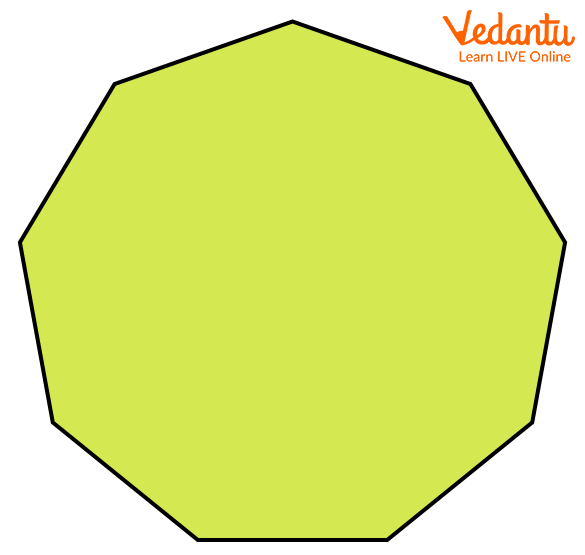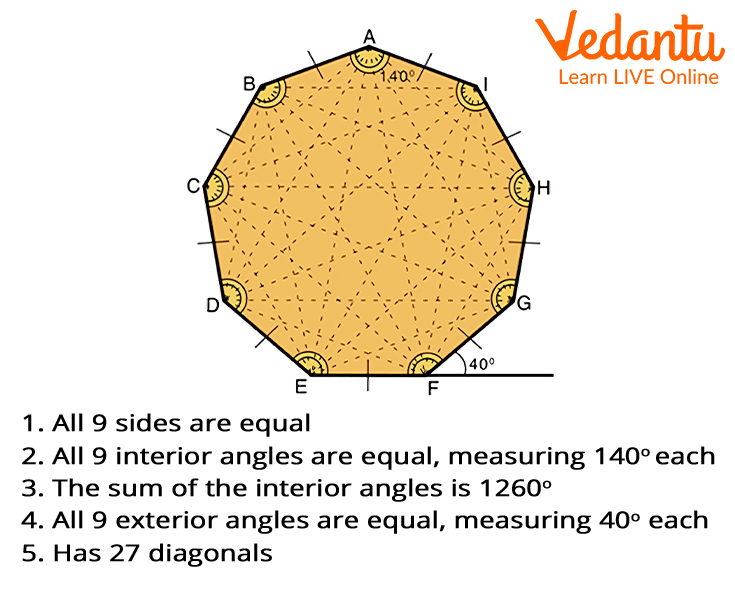Courses
Courses for Kids
Free study material
Free LIVE classes
More

# Nonagon: How is it in Shape?LIVE
Join Vedantu’s FREE Mastercalss

## Know in Detail about the Nine Sided Polygon

Does Geometry interest you? Then do you know how many sides a nonagon has? A nonagon is a kind of polygon that consists of nine sides and nine angles. The term ‘nonagon’ has been derived from the Latin word ‘nonus’, which means nine, and the Greek word ‘gon’, which means sides. It also has another name, ‘enneagon’, that is derived from the Greek word ‘enneagonon’, translating to nine corners. Despite ‘enneagon’ being a more appropriate term for this shape, ‘nonagon’ is more often used owing to the simplicity of the name and its convenience in use.Regular Nonagon

## Different Types of Nonagon

Based on their sides, angles, and vertices, nonagons can be categorised as follows:

• ccc: A regular nonagon has nine sides of equal length and nine interior angles, each of which measures 1400, and has exterior angles measuring 400 each. It has nine lines of symmetry and rotational equilibrium of order nine. All regular nonagons are convex.

• Irregular Nonagon: An irregular nonagon does not have all sides or all interior angles equal. However, the sum of all the nine interior angles measures up to 12600. Irregular nonagons can be both convex and concave.

• Convex Nonagon: A convex nonagon has all nine vertices pointing outwards. All interior angles of this type of nonagon measure less than or equal to 1800 and all diagonals are bounded by the closed figure. Convex nonagons can be both regular and irregular.

• Concave Nonagon: A concave nonagon has at least one vertex pointing inwards, having an interior angle that is greater than 1800. It has at least one diagonal lying outside the closed figure. Therefore, all concave nonagons are irregular.

## Properties of a Regular Nonagon

• All 9 sides are of equal length. For instance, in a nonagon ABCDEFGHI, AB = BC = CD = DE = EF = FG = GH = HI = IA.

• It comprises 9 interior angles, each measuring 1400. Hence, ∠ABC = ∠BCD = ∠CDE = ∠DEF = ∠EFG =∠FGH = ∠GHI = ∠HIA = ∠IAB.

• All 9 interior angles add up to 1260°. Therefore, ∠ABC + ∠BCD + ∠CDE + ∠DEF + ∠EFG + ∠FGH + ∠GHI + ∠HIA + ∠IAB = 12600.

• It consists of 9 exterior angles, each measuring 400.

• It has 27 diagonals, that is, AC, BD, CE, DF, EG, FH, and GI.Properties of a Regular Nonagon

Note: Even in an irregular nonagon, the sum of the interior angles will be 12600.

## Some Formulas Related to a Regular Nonagon

### Perimeter

The formula for the perimeter (P) of a regular nonagon ABCDEFGHI

P = 9a,

where a = length of each side of the nonagon, since all 9 sides are equal in a regular nonagon,  that is, a = AB = BC = CD = DE = EF = FG = GH = HI = IA.

Sample Problem: Determine the perimeter of a regular nonagon, each of whose sides measures 9 cm.

Solution: It is given that a = 9 cm.

Since Perimeter (P) = 9a,

Then, the required perimeter P = 9 x 9 cm

= 81 cm

### Area

The formula for determining the area of a regular nonagon ABCDEFGHI is as follows:

Area (A) = $\frac{9}{9}$ (a2cot $\frsc{π}{9}$ )

where a = side length = AB = BC = CD = DE = EF = FG = GH = HI = IA, and π = 1800.

### Angles

There are three major angle formulas concerned with regular nonagons - sum of interior angles, individual interior angles, and exterior angles. They can be elaborated as follows:

• Sum of Interior Angles - It is the sum of the measures of all the interior angles taken together in a nonagon. The formula for the sum of interior angles in a regular nonagon is given below:

Sum of the interior angles = (n-2) x 1800, where n = number of sides

In nonagon ABCDEFGHI, n = 9

Therefore,

Sum of the interior angles = (9 -2) x 1800

= 12600

• Individual Interior Angle - The measure of an individual interior angle in a regular nonagon can be obtained by dividing the sum of all the interior angles by the number of sides in the nonagon. The formula to obtain the value of an individual interior angle of a regular nonagon is given below:

One interior angle = (n-2) x 1800/n, where n = number of sides

In nonagon ABCDEFGHI, n = 9

Hence,

Sum of the interior angles = (9-2) x 1800/9

= 1400

• Exterior Angle - An exterior angle is an angle formed by any side of a nonagon and the extension of its adjacent side. The formula to find the value of an exterior angle is given below:

Exterior angle = 3600/n, where n = number of sides

In nonagon ABCDEFGHI, n = 9

Therefore,

Exterior angle = 3600/9

= 400

Nonagons may not be as commonly observed as other shapes, like circles, squares, and triangles, but their application in art and architecture is no less crucial. The following image is that of the US Steel Building, Pittsburgh, Pennsylvania. Although it may look like a triangular structure at a glance, taking a closer look will reveal its nonagonal aspects, that is, the 6 extra line segments ingrained in the magnificent architecture.US Steel Building, Pittsburgh, Pennsylvania

## Conclusion

To summarise the concept of nonagon, it is a two-dimensional figure (polygon) with nine sides and nine angles. It has 4 major types - regular, irregular, convex, and concave. Most of the properties and formulas are only applicable to regular nonagons, which have equal sides, and their interior and exterior angles are also equal, each measuring 1400 and 400, respectively. However, all nonagons have a total interior angle sum of 12600.

Last updated date: 22nd Sep 2023
Total views: 125.4k
Views today: 3.25k

## FAQs on Nonagon: How is it in Shape?

1. What are some real world examples of Nonagons?

The US Steel Building in Pittsburgh, Pennsylvania, is in the shape of an irregular nonagon. In daily life, we come across certain objects, like coins, drinking glasses, table-tops, etc., that are shaped like regular or irregular nonagons.

2. How many dimensions does a nonagon have?

A nonagon has two dimensions. Thus, it is a 2D figure or shape.